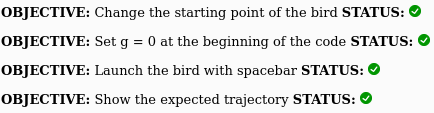# Bird Launcher!

If you have finished the Apollo moon landing activity, you can continue with this lab where we will configure the game to shoot a bird-shaped projectile.

From a physics perspective, bird launcher is essentially the same as Apollo moon landing except that there is no rocket thrust. Later on in this exercise it will be helpful to remember the equations for the x(t) and y(t) of a projectile that is launched into the air at t = 0

$$x(t ) = x_0 + v_{0x} t$$

$$y(t) = y_0 + v_{0y} t + \frac{1}{2} g t^2$$

where $g = -9.8$ instead of $-1.63$ because we will be on the earth instead of the moon. Remember that there is no acceleration in the x direction, which is why the equation for $x(t)$ is a bit simpler than the equation for $y(t)$

### Step 0. Open up a modified version of the Apollo moon landing code in an editor

Press play there to run the code. You will see something like this:By the end of this activity your goal is to have green check marks next to all the objectives like this:The steps below will hep you achieve all these objectives!

### Step 1. Look at the code

This code has been modified in a couple of ways from the Apollo moon landing code. Here it is:

function draw(){

// Update velocity
vx += ax*dt;
vy += ay*dt;

// Update location
x += vx*dt;
y += vy*dt;

// Check if arrow keys are pressed
if (keyIsDown(LEFT_ARROW)) {
v0x += -1.0;
}
if (keyIsDown(RIGHT_ARROW)) {
v0x += 1.0;
}
if (keyIsDown(UP_ARROW)) {
v0y += 1.0;
}
if (keyIsDown(DOWN_ARROW)) {
v0y += -1.0;
}

Fnety = mass*g;
ay = Fnety/mass;

// Draw axes and other stuff
// This will clear the screen and re-draw it
display();

drawBird(x,y,vx,vy,ax,ay);
drawForce(x,y,0,mass*g);

if (y < 0) {
drawText('Game Over!',width/3,height/2);
exit();
}

// Add more graphics here before the end of draw()

} // end draw()   DO NOT ADD ANYTHING AFTER THIS LINE!!!


What are the biggest differences from the Apollo moon landing program?

### Step 1. Change the starting point

After you click Duplicate, instead of the starting point being in the middle of the screen, configure it to start at the bottom left.

Change this:

x = 375;
y = 250;


to this:

x = 100;
y = 25;


The object should now start close to the bottom corner of the screen. Check to see that this works.

### Step 2a. Set g = 0 at the beginning of the code

We need to turn gravity off so we can turn it back on later. So the first step is to set g = 0 near the beginning of the code where the variables are all initialized.

At this point your program should behave like this

Notice that you can press the arrow keys to change the launch velocity, but the bird cannot move yet because gravity is turned off and there is nothing in the code to cause the bird to move at the launch velocity.

### Step 2b. Add code to launch the bird

Here is some code that you can use to launch the bird:

    if (keyIsPressed & key == ' ') { // spacebar
g = -9.8;
vx = v0x;
vy = v0y;
}


Once you do this the game should behave like this.

What do you notice about vx and vy? Do they behave differently after you launch the bird?

### Step 3: Show the expected trajectory

You should be able to calculate the trajectory of the bird from kinematic equation. In this step you will calculate this trajectory and draw it on the screen using drawPoint(xdraw,ydraw);

Somewhere after display(); add this code:


x0 = 100;
y0 = 25;

Npoints=1000;
for(i=1;i<=Npoints;i+=1)
{
t = (i-1)*dt;
xdraw = x0 + ?????;
ydraw = y0 + ?????;
drawPoint(xdraw,ydraw);
}


Fill in the ???? with the terms that give the right trajectory. The two equations at the beginning of this exercise should be a big help! The main problem is how to put this in the code and what to use for $v_{0x}$ and $v_{0y}$.

Advice #1: Expressions like t^2 won't work in this case (or in most other programming languages). Instead use t*t for $t^2$

Advice #2: Don't use vx or vy here because those variables will change after the object is launched. Instead use v0x and v0y

Advice #3: The formula for the trajectory includes earth's gravity. For code reasons, use -9.8 instead of g because g is initially zero.

Once you have figured this out the program should behave like this

### Challenge: Configure the code so spacebar only works the first time

You may notice that the bird only follows the parabolic trajectory if you tap the spacebar as briefly as you can. Configure the game so that pressing the spacebar only works the first time.

Once you have made this change, the game should behave like this

### How to get full credit on this programming lab!!!

1. Make sure the bird flies through the air like a projectile would (Steps 1-2)

2. Make sure that the drawn trajectory (dotted line) follows the path of the bird (Step 3)

It doesn't need to be perfect, but it should be pretty close (like this).

3. The challenge really is optional

If you want extra credit figure out how to make the spacebar only work the first time as discussed in the challenge. If you do not need extra credit then you can skip this if you want to.